Floquet theory

A theory concerning the structure of the space of solutions, and the properties of solutions, of a linear system of differential equations with periodic coefficients(1)

the matrixis periodic inwith period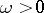and is summable on every compact interval in.

1) Every fundamental matrixof the system (1) has a representation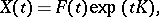(2)

called the Floquet representation (see ), where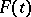is some-periodic matrix andis some constant matrix. There is a basisof the space of solutions of (1) such thathas Jordan form in this basis; this basis can be represented in the formwhere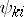are polynomials inwith-periodic coefficients, and the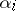are the characteristic exponents (cf. Characteristic exponent) of the system (1). Every component of a solution of (1) is a linear combination of functions of the form (of the Floquet solutions). In the case when all the characteristic exponents are distinct (or if there are multiple ones among them, but they correspond to simple elementary divisors), theare simply-periodic functions. The matricesandin the representation (2) are, generally speaking, complex valued. If one restricts oneself just to the real case, thendoes not have to be-periodic, but must be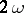-periodic.

2) The system (1) can be reduced to a differential equation with a constant matrix,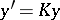, by means of the Lyapunov transformation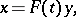(3)

whereandare the matrices from the Floquet representation (2) (see ). The combination of representation (2) together with the substitution (3) is often called the Floquet–Lyapunov theorem.

3) Let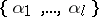be the spectrum of the matrix. For everysuch that,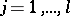, in view of (2) the spacesplits into the direct sum of two subspaces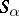and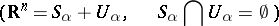such that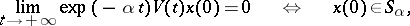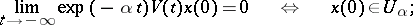hereis the fundamental matrix of (1) normalized at zero. This implies exponential dichotomy of (1) iffor any.